Final Exam Preparation

# Final Exam: Version A

## Questions from Chapters 1 to 3

1. Evaluate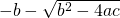if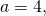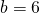and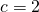.

For problems 2 and 3, solve for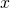.

1.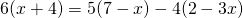2.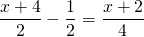3. Write an equation of the vertical line that passes through the point (−2, −3).
4. Find the distance between the points (−4, −2) and (2, 6).
5. Graph the relation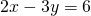.

For problems 7 and 8, find the solution set and graph it.

1.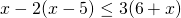2.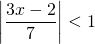In problems 9 and 10, set up each problem algebraically and solve. Be sure to state what your variables represent.

1. The time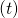required to empty a tank varies inversely to the rate of pumping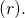If a pump can empty a tank in 45 minutes at the rate of 600 kL/min, how much time will it take the pump to empty the same tank at the rate of 1000 kL/min?
2. Find two consecutive odd integers such that their sum is 12 less than four times the first integer.

## Questions from Chapters 4 to 6

For problems 1–3, find the solution set of each system by any convenient method.

1.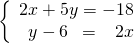2.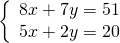3.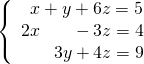For problems 4–6, perform the indicated operations and simplify.

1.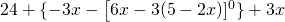2.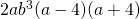3.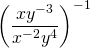For problems 7 and 8, factor each expression completely.

1.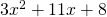2.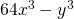3. A 50 kg mixture of two different grades of coffee costs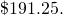If grade A is worth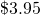per kg and grade B is worth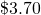per kg, how many kg of each type were used?
4. Kyra gave her brother Mark a logic question to solve: If she has 16 coins in her pocket worth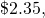and if the coins are only dimes and quarters, how many of each kind of coin does she have?

## Questions from Chapters 7 to 9

In problems 1–3, perform the indicated operations and simplify.

1.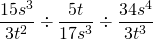2.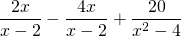3.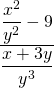For questions 4–6, simplify each expression.

1.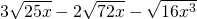2.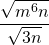3.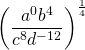For questions 7 and 8, solveby any convenient method.

1.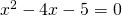2.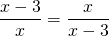In problems 9 and 10, find the solution set of each system by any convenient method.

1. The base of a right triangle is 6 cm longer than its height. If the area of this triangle is 20 cm2, find the length of both the base and the height.
2. Find three consecutive even integers such that the product of the first two is 8 more than six times the third number.

<a class=”internal” href=”/intermediatealgebraberg/back-matter/final-exam-prep-answer-key/”>Final Exam: Version A Answer Key